# Doing Subtraction in Excel

Feb 05, 2022It’s no secret that Excel can perform hundreds of calculations simultaneously, ranging from basic computations to complex statistical functions. Excel uses cell references in its formulas, so you can easily automate these functions. For young professionals, these basic skills mean landing a job, getting a promotion, or maybe just having an easier time at work. This article will teach you how to perform subtraction in Microsoft Excel in three ways:

##The First Way: Subtracting cell values

Step one: To get things started, we want to open Excel. We can do that by double-clicking on the desktop icon.Note that if you want to use an existing Excel document, double-click the one you need to open. (You can also do this in the file tab in Excel) Step Two:

###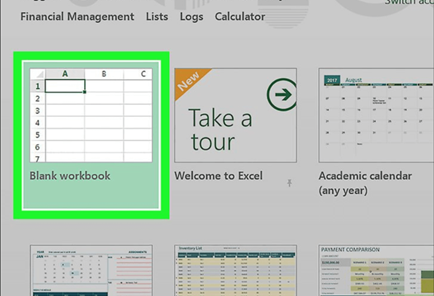Click "Blank workbook" (available on Windows) or "Excel workbook" (available on Mac). The button is at the top left of the Template window. Step Three: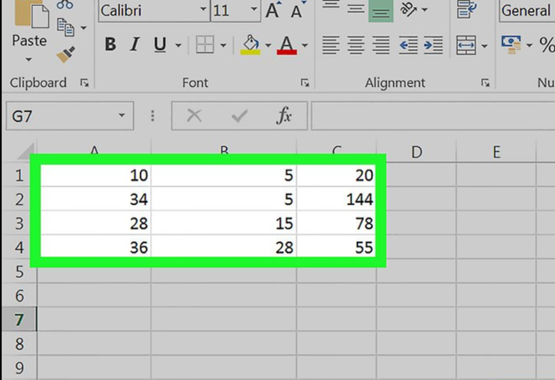Enter the data if needed. Do this by clicking on a cell, typing a number, and pressing ↵ Enter or ⏎ Return. Step Four: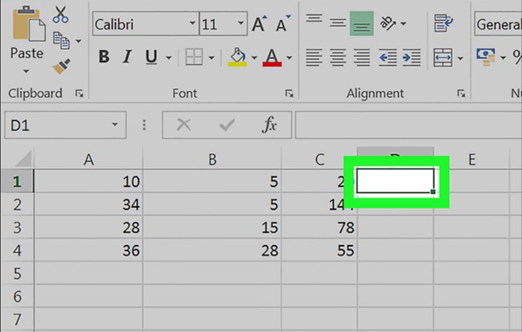Click on an empty cell. This selects the cell. Step Five: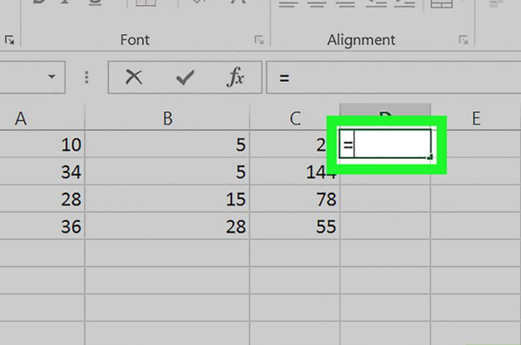With the cell selected, type in an equals symbol “=” into the cell. Type the value without the quotes. It would be best always to type "equals" before writing the formulas in Excel. This tells Excel that you want to start writing a formula. You can think of it as a reverse math equation. We add the equals symbol at the end of math equations where we want the answers to appear. Step Six: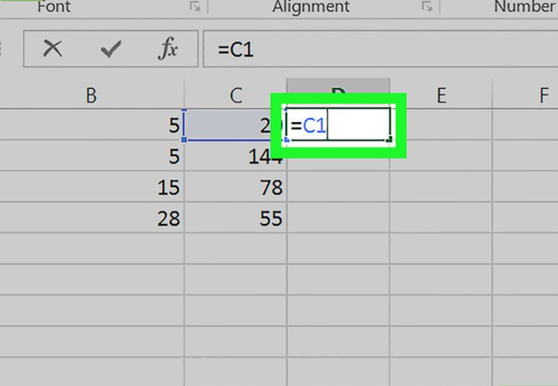Type a cell name. This is also called the cell reference in formulas. The cell whose name you type must be one from which you want to subtract the value or values of a cell or other cells. For example, you could type "C1" to specify the number in cell C1. Step Seven: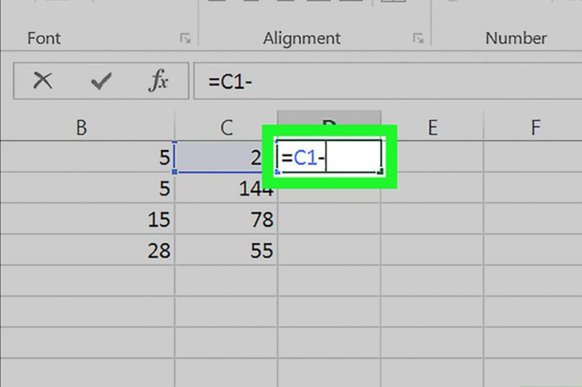Type in the dash or minus symbol "-"after the first cell reference. Step Eight: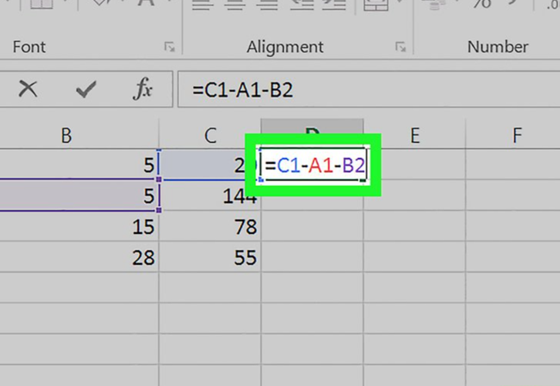Type the name of the other cells. This cell must be the cell you want to subtract from the first cell. You can repeat this process with multiple cells (e.g., "C1-A1-B2"). Step Nine: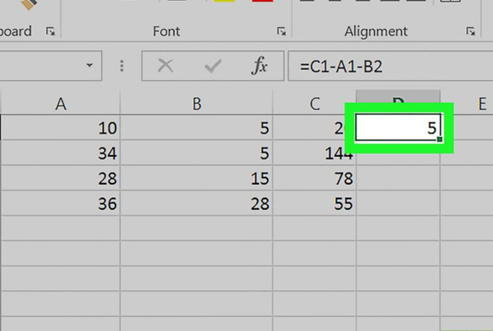Press ↵ Enter or ⏎ Return. Doing so will calculate the formula entered in the cell and replace it with the solution. You can click on the cell to display the original formula in the formula bar above the row of characters. Double-click on the cell or formula bar to edit the formula you've just made. You can also use the keyboard shortcut F2.

## The Second Way: Subtraction within a cell

Step One: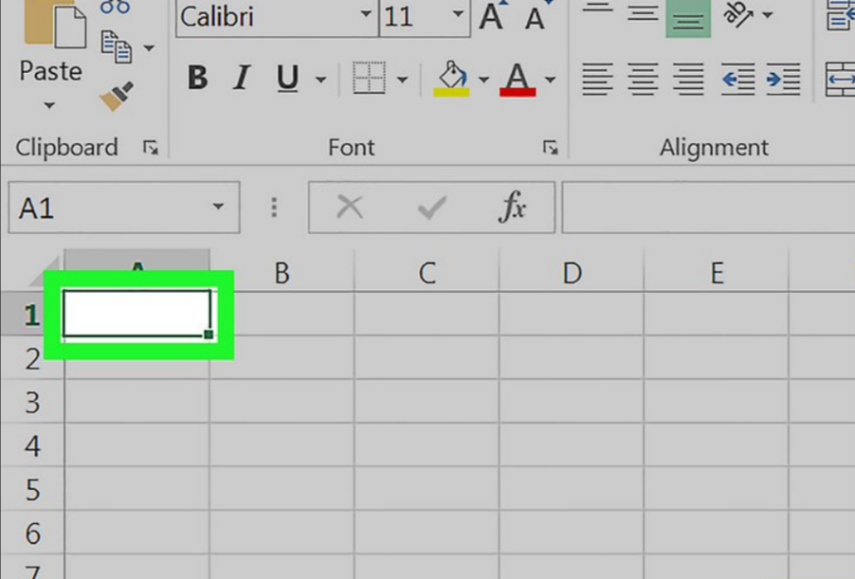Click on a cell. The cell you choose is not necessary unless you plan to create data with this workbook. Step Two: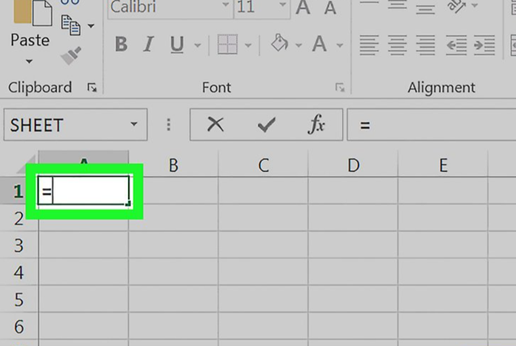Type “=” into the cell. Type the value without the quotes. This will prepare the cell for the formula. Step Three: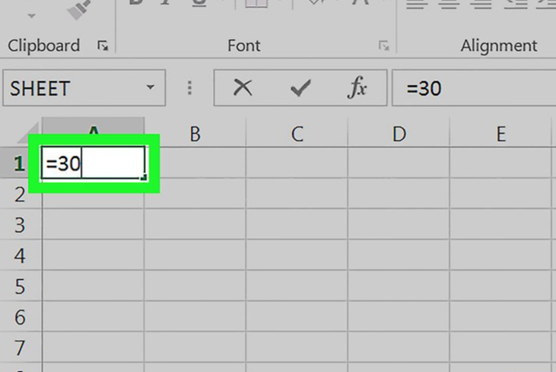Type the number you want to subtract from. The value will appear to the equal sign's right in the selected cell. For example, you could type your monthly income into this cell to set up your budget. Step Four: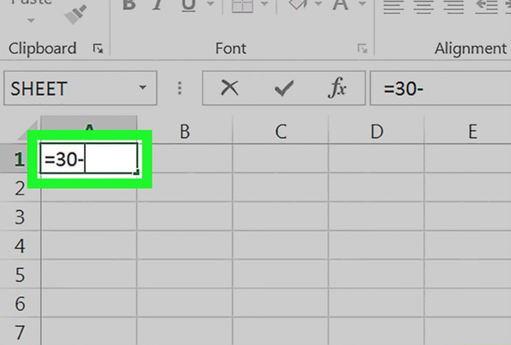Type (-) into the cell. The value will appear after the number you entered. If you subtract multiple values (X-Y-Z, for example), this step must be repeated after each successive number except for the last number. Step Five :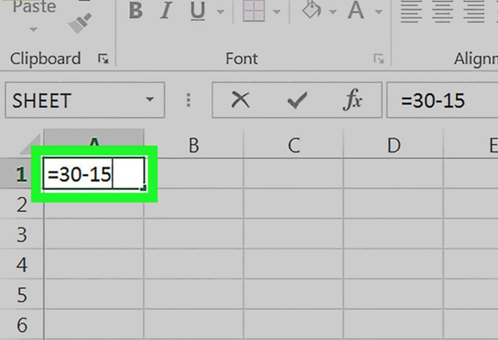Type the number you want to subtract from the first number. For example, you can write expenses in this cell if you calculate a budget. Step Six: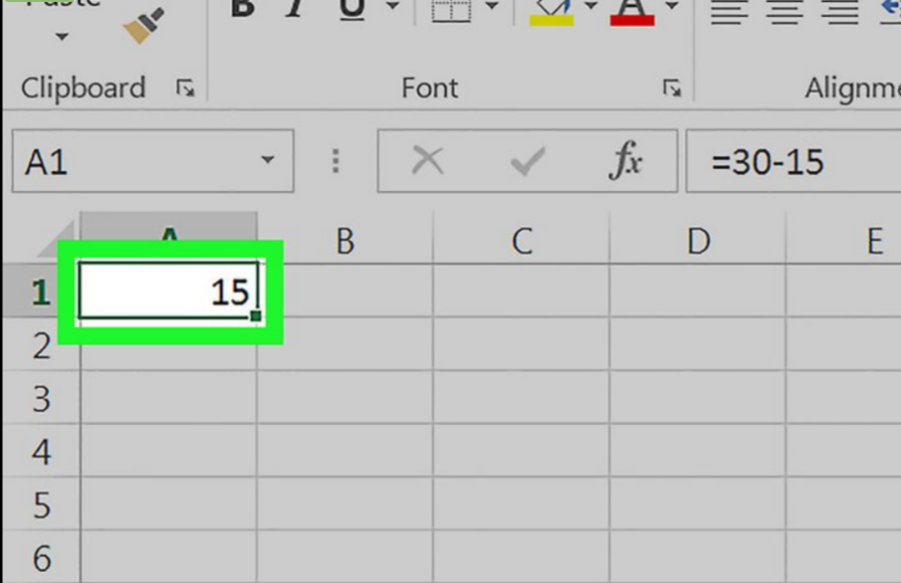Press ↵ Enter or ⏎ Return. Doing so will calculate the formula entered in the cell and replace it with the solution. You can click on the cell to display the original formula in the text bar above the row of characters.

## The Third Way: Column Subtract

Step One: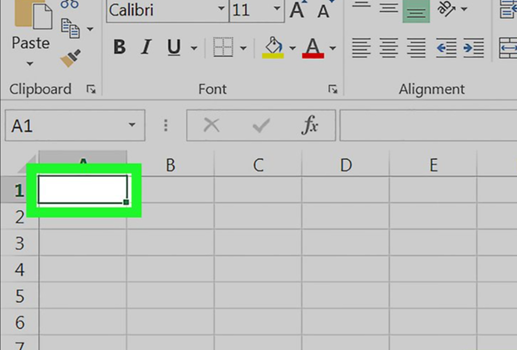Click on a cell. The cell you choose is not necessary unless you plan to create data with this workbook. Step Two: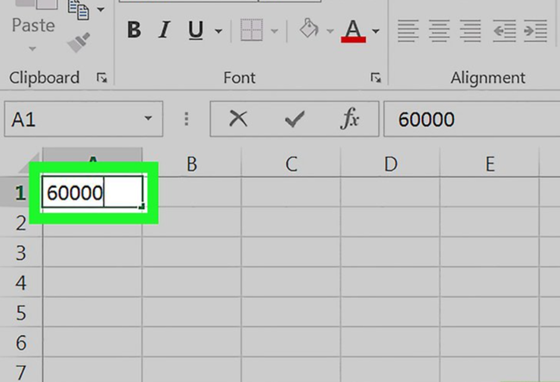Enter the critical value. This is the number from which the rest of the column will be selected. For example, you can write the annual salary in this field. Step Three: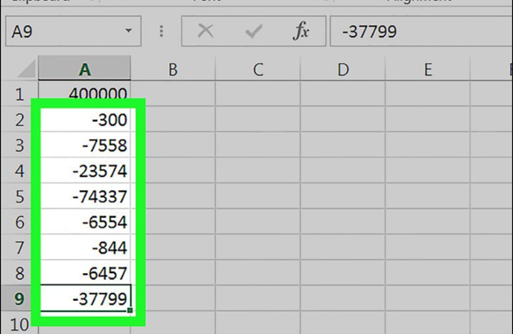Enter the deductions or the values you will be subtracted from the principal value in the lower cells. To do this, type a negative value for the number you want to subtract (e.g., "-300" if you want to subtract 300).

• One value should be written for each cell.
• Ensure that every number you type is in the same column as the main number.
• To apply this to the salary example, you'd probably type (-) followed by an expense in each cell.

Step Four: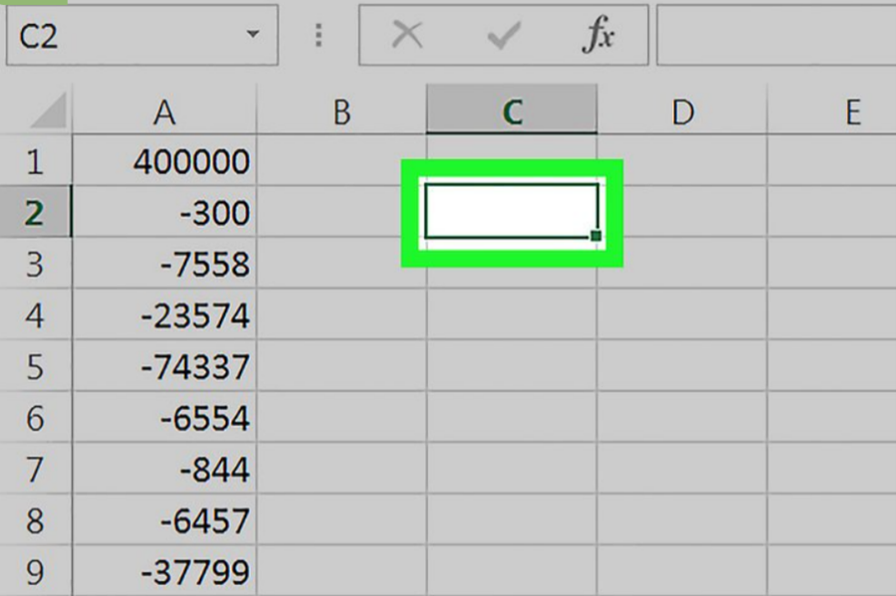Click on an empty cell. The cell does not need to be in the same column as the primary value this time. Step Five: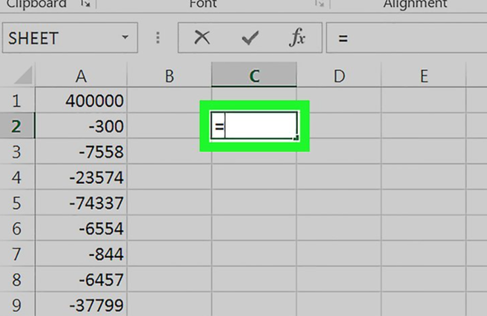Type "=" into the cell. Type the value without the quotes. Doing so prepares the cell for writing the formula. Step Six: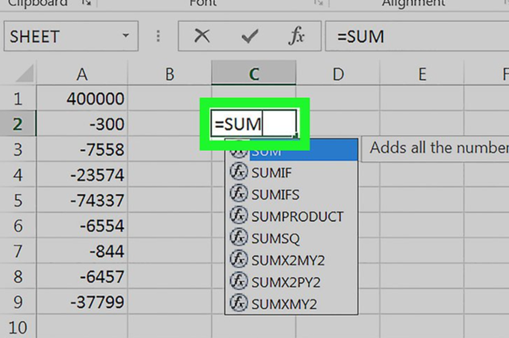Type SUM into the cell. The SUM command adds items together. There is no direct "subtract" command, so numbers are entered in a negative form.   Step Seven: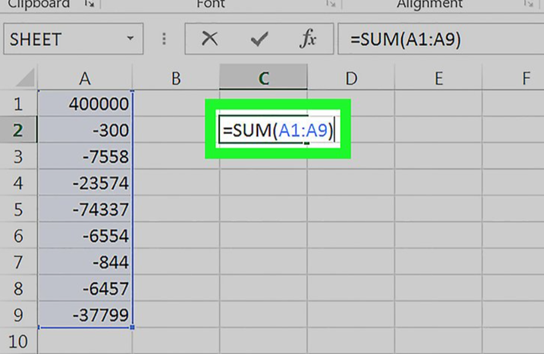Type (CellName:CellName) after SUM. This command adds all cells in a column from the first cell's value to the last cell's value. For example, if cell K1 is the base number and the cell with the last value is 'K10', you should type '(K1:K10)'. Step Eight: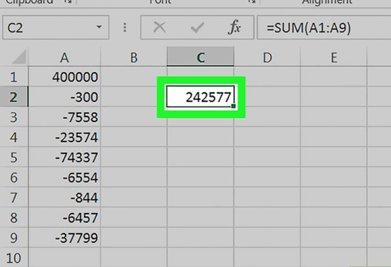Press ↵ Enter or ⏎ Return. Doing so will execute the formula in the selected cell, which will overwrite the formula with the final result.

## Some Quick Excel Tips

You can type formulas in Excel directly into a cell or the formula bar. You can also order formulas in Excel directly into a cell using functions or cell references (like column c or column b) and subtract multiple cells or dates using dynamic array formulas. Dates, times, and percentages can also be calculated by entering formulas in Excel. Columns in Excel are denoted by letters such as A, B., C, etc. Rows in Excel are numbered 1, 2, 3, etc. When you intersect a column and a description, you get a cell address in Excel, indicated by the column letter and row number; for example, the first cell will be set as A1. Sign up for the Simple Sheets website and get unlimited Excel ready-to-use templates.#### Want to Make Excel Work for You? Try out 5 Amazing Excel Templates & 5 Unique Lessons

We hate SPAM. We will never sell your information, for any reason.